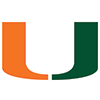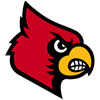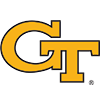DraftKings 2019 ACC Odds
-1250
0.08 to 1Clemson
70.3% implied probability

+700
7 to 1Virginia
9.5% implied probability

+1000
10 to 1Virginia Tech
6.9% implied probability

+2000
20 to 1Miami
3.6% implied probability

+2000
20 to 1Pittsburgh
3.6% implied probability

+2400
24 to 1Wake Forest
3.0% implied probability

+4000
40 to 1North Carolina State
1.9% implied probability

+10000
100 to 1Louisville
0.8% implied probability

+25000
250 to 1Duke
0.3% implied probability

+50000
500 to 1Georgia Tech
0.2% implied probability